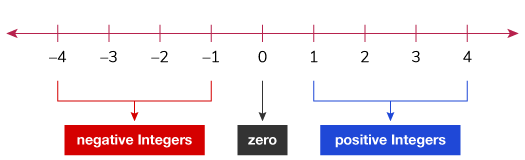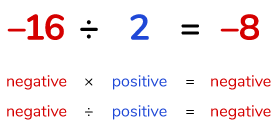# Multiplication and Division of Integers

Go back to  'Integers'

## Introduction to Multiplication and Division of Integers

We have all learnt about multiplication and division for whole numbers. But what happens when negative integers are involved? The rules of multiplication and division is slightly different because there are different cases involved. We will learn more about it in the detail.

## The idea behind multiplication and division of integers

• Multiplication of whole or natural numbers involves adding a particular number a given number of times. For example, 4 x 3 is nothing but adding 4 three times.
• Dividing whole or natural numbers is distributing a quantity into equal groups.
• With division, you start with a larger number and break it down multiple times till you are left with the smallest number possible

### What are Integers?

• An integer by definition is a whole number that can be either positive, negative or zero (but cannot be a fraction). Integers are represented by Z and are written as;

$$\text{Z} = \text{{…, –3, –2, –1, 0, 1, 2, 3, …}}$$

• Integers cannot be $$-1.25,$$ $${2 \over 3},$$ $$-589.332$$## Multiplication of Integers

• We know that multiplication is nothing but repetitive addition.
• When we come to the case of integers, the following cases must be taken into account:
1. Multiplying 2 positive numbers
2. Multiplying 2 negative numbers
3. Multiplying 1 positive and 1 negative number

When you multiply two integers the same sign;

Positive x positive = positive = 2 x 5 = 10

When you multiply two integers with two negative signs;

Negative x Negative = Positive = –2 x –3 = 6

When you multiply two integers with one negative sign and one positive sign;

Negative x positive = negative = –2 x 5 = –10

 Types of Integers Result Example Both Integers Positive Positive 2 x 5 = 10 Both Integers Negative Positive –2 x –3 = 6 1 Positive and 1 Negative Negative –2 x 5 = –10

Now try out the following game. Move the cannon with the arrow keys and to shoot click on the cannon.

Author: Aleksandra-Maria Vuković

## Division of Integers

Just like multiplication, division of integers also involve the same cases.

• Dividing 2 positive numbers
• Dividing 2 negative numbers
• Dividing 1 positive and 1 negative number

When you divide two integers with the same sign;

Positive ÷ positive = positive 16 ÷ 8 = 2

When you divide two integers with two negative signs;

Negative ÷ negative = positive = –16 ÷ –8 = 2

When you divide two integers with one negative sign and one positive sign;

Negative ÷ positive = negative = –16 ÷ 8 = –2

 Types of Integers Result Example Both Integers Positive Positive 16 ÷ 8 = 2 Both Integers Negative Positive –16 ÷ –8 = 2 1 Positive and 1 Negative Negative –16 ÷ 8 = –2To sum it all up, to make everything easy, the two most important things to remember whether you are multiplying or dividing 2 integers;

1. When the signs are different, the answer is always negative.
2. When the signs are the same, the answer is always positive.

Now, test what you have learnt till now by trying out the exercise given below:

Author: Sébastien Vieilhescazes

## Problems faced during multiplication and division of integers

• Most students are confused when there are more than 2 integers with different signs that have to be either multiplied or divided. The confusion of what the resultant sign is is the big question.
• To make it easier the rules of multiplication and division of integers is of utmost importance.
• Work the equation from left to right, apply the rules to each sign and makes sure that mistakes are not be made.

For example

### Division

(–20) ÷ (–5) ÷ (–2) =?

The solution

Step 1: (–20 ÷ –5) ÷ (–2)
Step 2: (10) ÷ (–2)
10 is positive as negative ÷ negative = positive
Step 3: –5
Positive ÷ negative = negative
Hence (–20) ÷ (–5) ÷ (–2) = –5

### Multiplication

(–6) x (–4) x (3) =?

The solution

Step 1: (–6 x –4) x (3)
Step 2: 24 x 3
24 is positive as negative x negative is positive
Step 3: 72
Hence (–6) x (–4) x (3) = 72

Note: Another easy method to do the above problems are given below.

• Multiply or divide without the negative sign.
• The sign of the final answer can be determined by the number of negative sign.
• If the total number of negative signs is even, the final answer will be positive.
• If the total number of negative signs is odd, the final answer will be negative.

Example:

Evaluate $$( -6 ) \times ( -4 ) \times (3)$$.

Solution:

Step 1: Multiply without the signs.

$$( 6 ) \times ( 4 ) \times ( 3 ) = 72$$

Step 2: Count the number of signs.

In this case it is 2.

So, there are even number of negative sign.

This means the resultant sign will be positive.

So, the final answer to the problem is $$72$$

## Activity:

 Activitiy Name Sub_MultiplicationDivisionIntegers_Activity Item Name 1 Sub_MultiplicationDivisionIntegers_Item1 Item Name 2 Sub_MultiplicationDivisionIntegers_Item2 Item Name 3 Sub_MultiplicationDivisionIntegers_Item3 Item Name 4 Sub_MultiplicationDivisionIntegers_Item4 Item Name 5 Sub_MultiplicationDivisionIntegers_Item5

### 1. What is the rule of division of integers?

Ans. The rules for division of integers are given below:

• Positive ÷ positive = positive
• Negative ÷ negative = positive
• Negative ÷ positive = negative

### 2. How do you multiply integers?

• Multiply without the negative sign.
• If both the integers are negative or both are positive, the final answer will be positive.
• If one integer is positive and the other is negative, the final answer will be negative.

### 3. How do you multiply multiple integers?

Ans.

• Multiply without the negative sign.
• The sign of the final answer can be determined by the number of negative sign.
• If the total number of negative signs is even, the final answer will be positive.
• If the total number of negative signs is odd, the final answer will be negative.

### 4. What are the four rules for multiplying integers?

Ans.

• Rule 1: Positive x Positive = Positive
• Rule 2: Positive x Negative = Negative
• Rule 3: Negative x Positive = Negative
• Rule 4: Negative x Negative = Positive

### 5. How do you multiply a positive and negative integer?

Ans.

• Multiply without the negative sign.